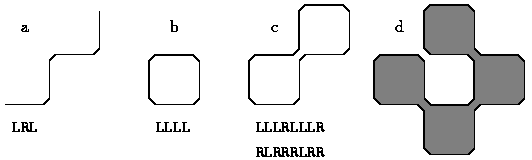## Fence FactoryFigure 1: Four fences and some of their letter strings (joints not to scale)

A factory makes a modular fence system. The fence sections are one unit long and may be linked together using a separate joint. A joint always links two sections. Unfortunately, the machine that makes straight joints has broken down. The machine for right-angled (90°) joints still works, and can make left-handed and right-handed joints.

The factory's computer scientist is asked to work out what shapes can be made with right-angled joints only. The computer scientist decides to describe a fence by a string of letters. Each letter tells whether the next section is joined to the left (`L`) or the right (`R`).

The string `LRL` describes a zigzag, consisting of 4 sections (Figure 1a; the joint `cutoff' is greatly exaggerated). The string `LLLL` describes a closed square (Figure 1b). Some strings describe impossible shapes that intersect themselves, e.g. `RLLLL`.

From now on we consider only strings describing possible shapes that are closed.

A closed shape can be encoded in several ways, depending on where you start and the direction you go. For example, the strings `LLLRLLLR` and `RLRRRLRR` describe the same closed shape (Figure 1c).

Write a program that inputs a string describing a closed shape, and answers the following questions for this shape:

1. How large is the enclosed area, in square units? Space that can be reached from outside by squeezing in between two joints is not considered enclosed (see Figure 1d: the enclosed area is shaded).
2. Which strings describe this shape?

### Input Format

The input consists of one line containing a single string and nothing else. A string consists of at least 4 and at most 50 letters.

### Output Format

The output should consist of at least two lines. On the first line is a single positive integer: the area enclosed by the shape (Subtask A). The next lines each contain a string describing the shape (Subtask B). These strings may be given in any order, but they must all be different.

### Sample Input

```LLLRLLLR
```

### Sample Output

```2
LLLRLLLR
LLRLLLRL
LRLLLRLL
RLLLRLLL
RRRLRRRL
RRLRRRLR
RLRRRLRR
LRRRLRRR
```

Point Value: 15 (partial)
Time Limit: 1.00s
Memory Limit: 8M